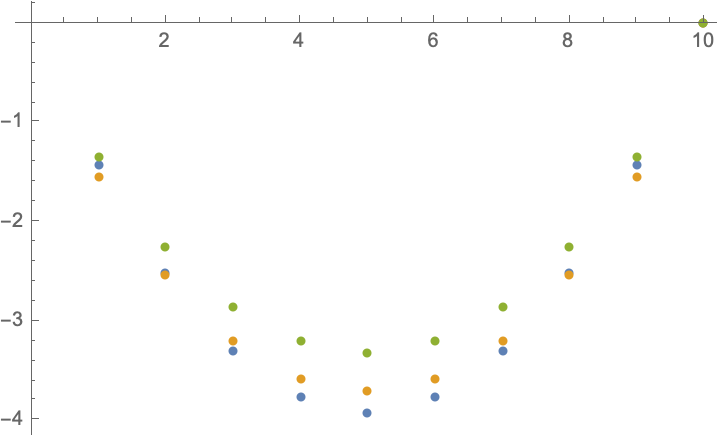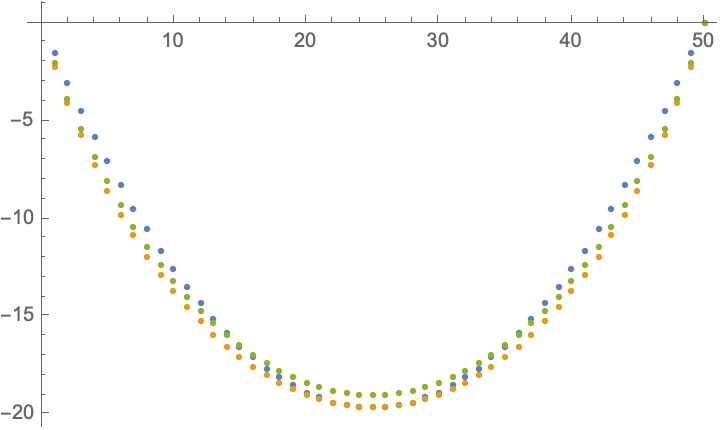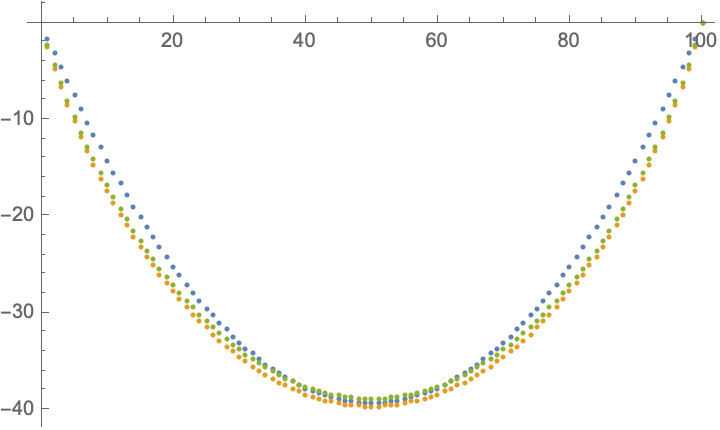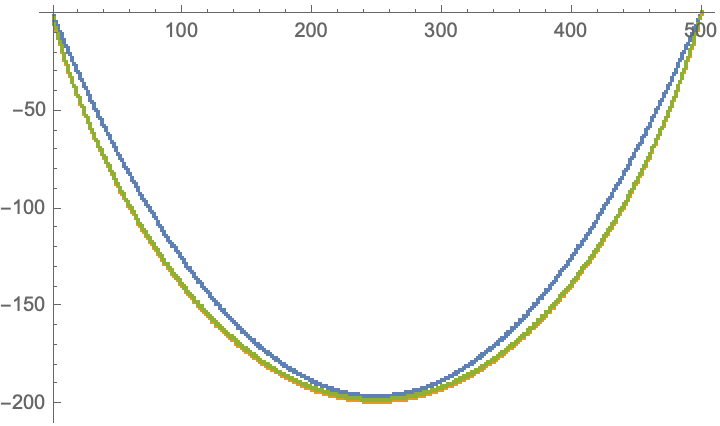# What is the expected value of the sum of the k (out of a set of n) smallest normal random variables?

Given $$n$$ independent normally distributed random variables $$X_1,X_2,...,X_n \sim N(\mu,\sigma)$$. For any $$k\leq n$$, let $$X_{(k)}$$ be the k-th order statistics (i.e., the k-th smallest value). What is the expected sum $$\mathbb{E}[\sum_{r=1}^k X_{(r)}]$$?

Closed-form expressions for arbitrary $$n,k$$ are not available. For small $$k$$ you can use an integral expression to evaluate $$\mathbb{E}[\sum_{r=1}^k X_{(r)}]=\sum_{r=1}^k\mathbb{E}[X_{(r)}]$$, with $$\mathbb{E}[X_{(r)}]=\frac{n!}{(r-1)!(n-r)!}\int_{-\infty}^\infty[1-\Phi(x)]^{r-1}[\Phi(x)]^{n-r}\frac{x}{\sqrt{2\pi}}e^{-x^2/2}\,dx.$$ (Here $$\Phi(x)$$ is the CDF of the normal distribution.)
For example, for $$n=5$$ and $$k=1,2,\ldots n$$, you would find $$\mathbb{E}\left[\sum_{r=1}^k X_{(r)}\right]=\frac{5}{2\pi^3}\times\left(\pi -6 \arctan\sqrt{2},- 3\pi +6 \arctan\sqrt{2},- 3\pi +6 \arctan\sqrt{2},\pi -6 \arctan\sqrt{2},0\right).$$
The large-$$n$$ asymptotics is given in terms of the functional inverse $${\rm erf}^{-1}$$ of the error function, $$\mathbb{E}\left[\sum_{r=1}^k X_{(r)}\right]\rightarrow\sqrt{2}\sum_{r=1}^k\text{erf}^{-1}\left(\frac{2r}{n+1}-1\right),\;\;\text{for}\;\;n\rightarrow\infty,$$ which is cumbersome to evaluate. As an alternative simple formula for large $$n$$ I can offer the parabolic approximation $$\mathbb{E}\left[\sum_{r=1}^k X_{(r)}\right]\approx -\frac{\pi k}{2 n} (n-k).$$
The plots compare the exact result (gold) with the parabolic approximation (blue) and the $$\text{erf}^{-1}$$ asymptote (green) for $$n=10$$, $$50$$, $$100$$, and $$500$$. As you can see, the parabolic approximation is accurate within 10% even for relatively small $$n$$.